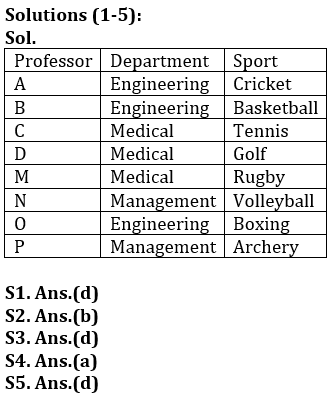Latest Banking jobs   »

# Reasoning Quiz For Bank of Baroda AO 2023-20th March

Directions (1-5): Study the following information carefully and answer the questions given below.
Eight professor- A, B, C, D, M, N, O, and P are working in three departments Engineering, Medical, and Management of an Institute with at least two of them work in each department and not more than three persons work in same departments. Each of them likes a different sports Golf, Volleyball, Archery, Boxing, Tennis, Rugby, Basketball and Cricket but not necessarily in the same order.
P likes Archery and he works in Management department with only N. B and M do not work in the same department. O likes Boxing and does not work in medical department. M and C works together but not in engineering department. D works with C in the same department and likes Golf. B likes Basketball and none of his colleagues in the department likes Tennis and Rugby. N likes Volleyball and M does not like Tennis.

Q1. Who among the following works same department as B?
(a) C
(b) D
(c) M
(d) O
(e) P

Q2. Which of the following sport likes by M?
(a) Cricket
(b) Rugby
(d) Volleyball
(e) Tennis

Q3. Four of the following five form a group, which among the following does not belong to that group?
(a) D-Medical
(b) C-Tennis
(c) N-Management
(e) O-Engineering

Q4. Who among the following likes tennis?
(a) C
(b) A
(c) M
(d) O
(e) N

Q5. Which of the following group of persons work in the same department?
(a) N-O
(b) P-C
(c) A-M
(d) O-B
(e) D-A

Directions (6-10): The following questions are based on the five words in each of three letters given below.
TRA  PTQ  YTV  WEN  CBN

Q6. If all the letters in each of the words are arranged in alphabetical order within the word, then how many meaningful words will be formed?
(a) One
(b) Two
(c) Three
(d) More than three
(e) None

Q7. If the first letter of each word replaces by succeeding letter in English alphabet, then how many words have more than one vowel?
(a) Three
(b) Two
(c) One
(d) More than three
(e) None

Q8. If all the words are arranged in alphabetical order, then how many words remains at the same position?
(a) None
(b) One
(c) Two
(d) Three
(e) More than three

Q9. If first and last letter of each word are interchanged their position, then how many meaningful words are formed?
(a) One
(b) Two
(c) Three
(d) More than three
(e) None

Q10. If all words are arranged in alphabetical order from left after interchanging first and second letter of each word, then which of the following word is 3rd from right?
(a) YTV
(b) TRA
(c) WEN
(d) CBN
(e) None of these

Directions (11-15): Following questions are based on the five three-digit numbers given below.

947  376  694  739  863

Q11. If all the digits in each of the numbers are arranged in increasing order within the number, then, which of the following number will become the lowest in the new arrangement of numbers?
(a) 947
(b) 863
(c) 739
(d) 694
(e) 376

Q12. If all the numbers are arranged in ascending order from left to right then, which of the following will be the sum of all the three digits of the number which is 2nd from the right in the new arrangement?
(a) 18
(b) 19
(c) 15
(d) 16
(e) None of these

Q13. What will be the difference, when third digit of the 3rd lowest number is multiplied with the second digit of the highest number and third digit of the 2nd highest number is multiplied with the second digit of the lowest number?
(a) 21
(b) 20
(c) 15
(d)16
(e) None of these

Q14. If the positions of the second and the third digits of each of the numbers are interchanged then, how many even numbers will be formed?
(a) None
(b) One
(c) Two
(d) Three
(e) Four

Q15. If one is added to the second digit of each of the numbers and one is subtracted to the third digit of each number then, how many numbers thus formed will be divisible by three in new arrangement?
(a) None
(b) One
(c) Two
(d) Three
(e) Four

SolutionsSolutions (6-10)
S6. Ans(a)
Sol. ART
S7. Ans(c)
S8. Ans(c)
S9. Ans(b)
S10.Ans(b)

Solution (11-15):
S11.Ans(e)
S12.Ans(e)
S13.Ans(c)
S14.Ans(c)
S15.Ans(a)## FAQs

### What is the selection process of Bank of Baroda AO 2023?

The selection process of Bank of Baroda AO consists of Online exam and Interview

#### Congratulations!Union Budget 2023-24: Free PDF